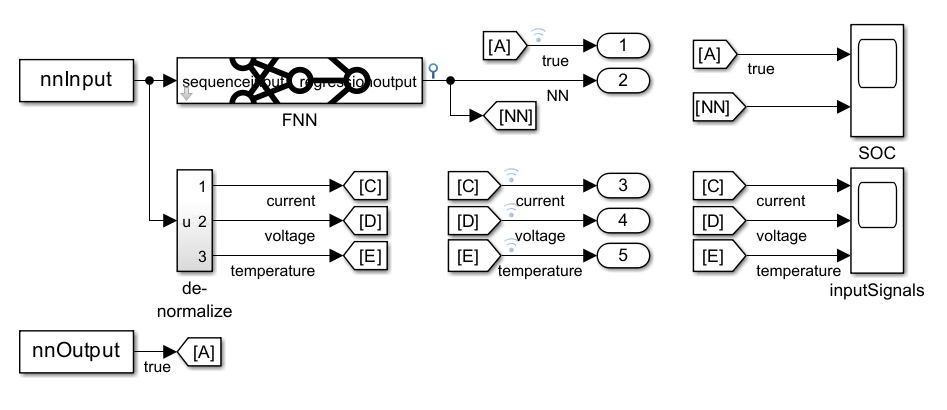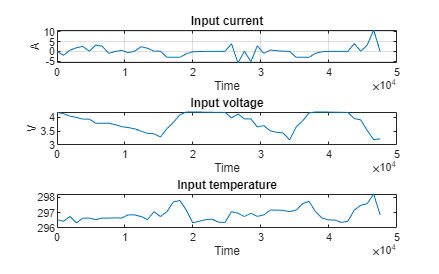# Battery State of Charge Estimation in Simulink Using Deep Learning Network

This example shows how to use a feedforward deep learning network inside a Simulink® model to predict the state of charge (SOC) of a battery. You include the network inside the Simulink model by using a Predict block, which predicts the SOC at every simulation step.

Battery SOC is the level of charge of an electric battery relative to its capacity measured as a percentage. SOC is critical information for the vehicle energy management system and must be accurately estimated to ensure reliable and affordable electrified vehicles. Methods based on the Kalman filter (EKF) algorithm are the traditional approaches to this problem but they usually require precise parameters and knowledge of the battery composition as well as its physical response. In contrast, using neural networks is a data-driven approach that requires minimal knowledge of the battery or its nonlinear behavior .

This example uses the preprocessed data set `LG_HG2_Prepared_Dataset_McMasterUniversity_Jan_2020` from . The example uses a trained feedforward neural network to predict the SOC of a Li-ion battery, given time series data representing various features of the battery such as voltage, current, temperature, average voltage, and average current. For an example showing how to train the network, see Predict Battery State of Charge Using Deep Learning.

### Simulink Model to Predict SOC

Open the Simulink model `BatterySOCSimulinkEstimation.slx`.

```BatterySOCSimulinkEstimation_ini; modelName = 'BatterySOCSimulinkEstimation'; open_system(modelName);```The model uses two From Workspace blocks to load the predictors for the trained network and the target SOC from the test data, a Predict block from the Deep Learning Toolbox™ library, and two `Scope` blocks to show the predicted output and the input signals.

The Predict block predicts responses for the data at the input by using the trained network that you specify using the block parameters. The block accepts an input signal with a dimensionality of the input layer of the neural network and outputs the prediction.

An EKF Subsystem implements the extended kalman filter algorithm to recursively estimate the state of charge at each simulation step.

### Run Simulation

To predict the state of charge of the battery and verify the efficiency of the prediction, run the simulation.

`sim('BatterySOCSimulinkEstimation');`

You can integrate this system within a larger framework, for example, in a battery management system that continuously monitors the status of the battery and enacts precautionary measures if the battery operates outside its safe operating area.

### Plot Input

To plot the inputs, use the `soc_estimation_plot_inputs.m` script.

`BatterySOCSimulinkEstimation_plot_inputs;`### Plot and Analyze Output

To analyze the performance of the network, compare the results of predictions obtained from the network and the EKF with the test data.

To plot the outputs, use the `soc_estimation_plot_outputs.m` script.

`BatterySOCSimulinkEstimation_plot_outputs;`The plot shows the neural network predictions of the SOC over time. The network predictions are close to the SOC values obtained from the test data. The network predicts the state of charge with an accuracy of 3 within a temperature range between –10˚ C and 25˚ C.

### References

 Kollmeyer, Phillip, Carlos Vidal, Mina Naguib, and Michael Skells. “LG 18650HG2 Li-Ion Battery Data and Example Deep Neural Network XEV SOC Estimator Script.” Mendeley, March 5, 2020. https://doi.org/10.17632/CP3473X7XV.3.